# Convexity and Quantum Physics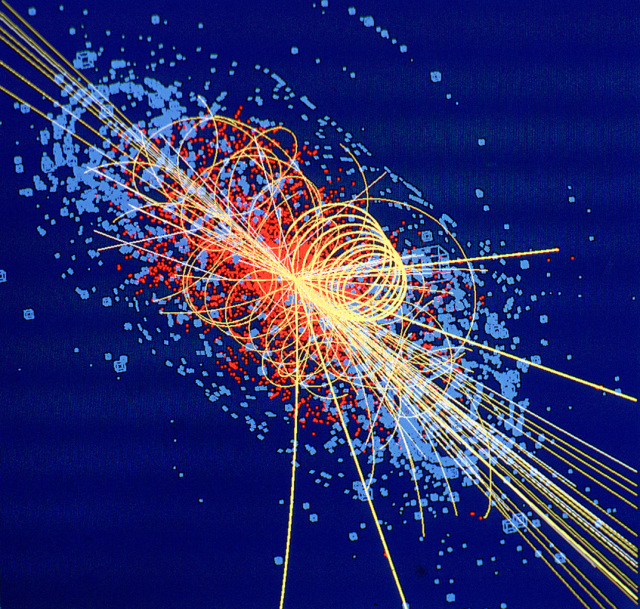February 15, 2022# Quantum Mechanics# Ingredients of Quantum Mechanics

• Vector space of states ($$|\psi\rangle$$)
• Positive-semidefinite inner product: $$\langle \psi | \psi \rangle \ge 0$$
• Hermitian observables
• Hamiltonian (Hermitian generator of time evolution)
• $U(t) = e^{-i H t}$
• Ground state $$|\Omega\rangle$$ minimizing $$\langle \Omega | H| \Omega\rangle$$

Questions we can ask
• Ground state properties
• Thermal properties
• Time-dependent behavior (near or far from equilibrium)

# Example: Harmonic Oscillator

$\hat H = \frac{1}{2} \hat x^2 + \frac{1}{2} \hat p^2$

Remember: $${\color{green}\langle \Psi|\color{blue}|\Psi\rangle}\ge 0$$

Of course $${\color{green}\langle 0 | x \color{blue}x | 0 \rangle} \ge 0$$ and $${\color{green}\langle 0 | p \color{blue}p | 0 \rangle} \ge 0$$. So: $$\langle \hat H \rangle \ge 0$$.

We can do better, by using the uncertainty principle: $$[x,p] = i\hbar$$.

Take $$|\Psi\rangle = (\hat x+i\hat p)|0\rangle$$. $\langle (\hat x-i\hat p) (\hat x+i\hat p) \rangle = \langle \hat x^2 \rangle +i\langle[\hat x,\hat p]\rangle +\langle \hat p^2 \rangle \ge 0$

This is the classic proof that $$\langle \hat H \rangle \ge \frac \hbar 2$$ for the harmonic oscillator.

# Example: A Spin

$H = - \mu \sigma_x$Define $$|\Psi\rangle = (1 + \sigma_x)|0\rangle$$ $\langle \Psi | \Psi\rangle \ge 0 \Longrightarrow \langle 2 + 2\sigma_x \rangle \ge 0 \Longrightarrow \langle \sigma_x \rangle \ge -1$

So $$\langle H\rangle \ge -\mu$$ (as expected!)

Both bounds happen to be tight. This won't always happen.

# Being More Systematic

Let's move from states to operators!

$\color{green} \langle \mathcal O^\dagger \mathcal O \rangle \ge 0$

Consider a basis of operators $$\mathcal O_1,\ldots\mathcal O_N$$. We can build a matrix $M = \left(\begin{matrix} \langle \mathcal O_1^\dagger \mathcal O_1 \rangle & \langle \mathcal O_1^\dagger \mathcal O_2\rangle & \cdots \\ \langle \mathcal O_2^\dagger \mathcal O_1 \rangle & \langle \mathcal O_2^\dagger \mathcal O_2\rangle & \cdots \\ \vdots & \vdots & \ddots \end{matrix}\right)$

The matrix $$M$$ is positive semi-definite!

Proof: for any vector $$v$$, consider the operator $$\mathcal O = \sum_i v_i \mathcal O_i$$. $v^\dagger M v = \langle (\vec v \cdot \mathcal {\vec O})^\dagger (\vec v \cdot \mathcal {\vec O}) \rangle = \langle \mathcal O^\dagger \mathcal O \rangle \ge 0$

With a complete set of operators, this sums up the entire positivity axiom.

# Semidefinite Programs

This is a specific case of a semi-definite program.

Let $$C$$ be an $$N \times N$$ matrix. We wish to find a positive semi-definite matrix $$X$$ $\text{minimizing }\;\mathrm{Tr}\;CX$ subject to linear constraints on the matrix elements of $$X$$.

In our context, $$C$$ specifies the Hamiltonian, and the linear constraints follow from the commutation relations.

Example: $$\langle xp\rangle - \langle px\rangle = i\hbar$$

# One Spin, Again

Here's a basis of operators: $$\{1, \sigma_x, \sigma_y, \sigma_z\}$$. (In fact this basis is complete.)

$\color{green} \left(\begin{matrix} 1 & \langle \sigma_x \rangle & \langle \sigma_y \rangle & \langle \sigma_z \rangle\\ \langle \sigma_x \rangle & 1 & i \langle \sigma_z \rangle & -i \langle \sigma_y \rangle\\ \langle \sigma_y \rangle & -i \langle \sigma_z \rangle & 1 & i\langle \sigma_x \rangle\\ \langle \sigma_z \rangle & i\langle \sigma_y \rangle & -i \langle \sigma_x \rangle & 1 \end{matrix}\right)\succeq 0$

Note that any assignment of expectation values obeying the above constraint corresponds to some density matrix $$\rho$$.

For $$H = -\mu \sigma_x$$, only the upper-left $$2\times 2$$ minor is needed. $\left|\begin{matrix} 1 & \langle \sigma_x \rangle\\ \langle \sigma_x \rangle & 1 \end{matrix}\right|\ge 0 \Longrightarrow 1 - \langle \sigma_x \rangle^2 \ge 0$

# Visualizing The One-Spin SDP

$\left(\begin{matrix} 1 & \langle \sigma_x \rangle\\ \langle \sigma_x \rangle & 1 \end{matrix}\right)\succeq 0$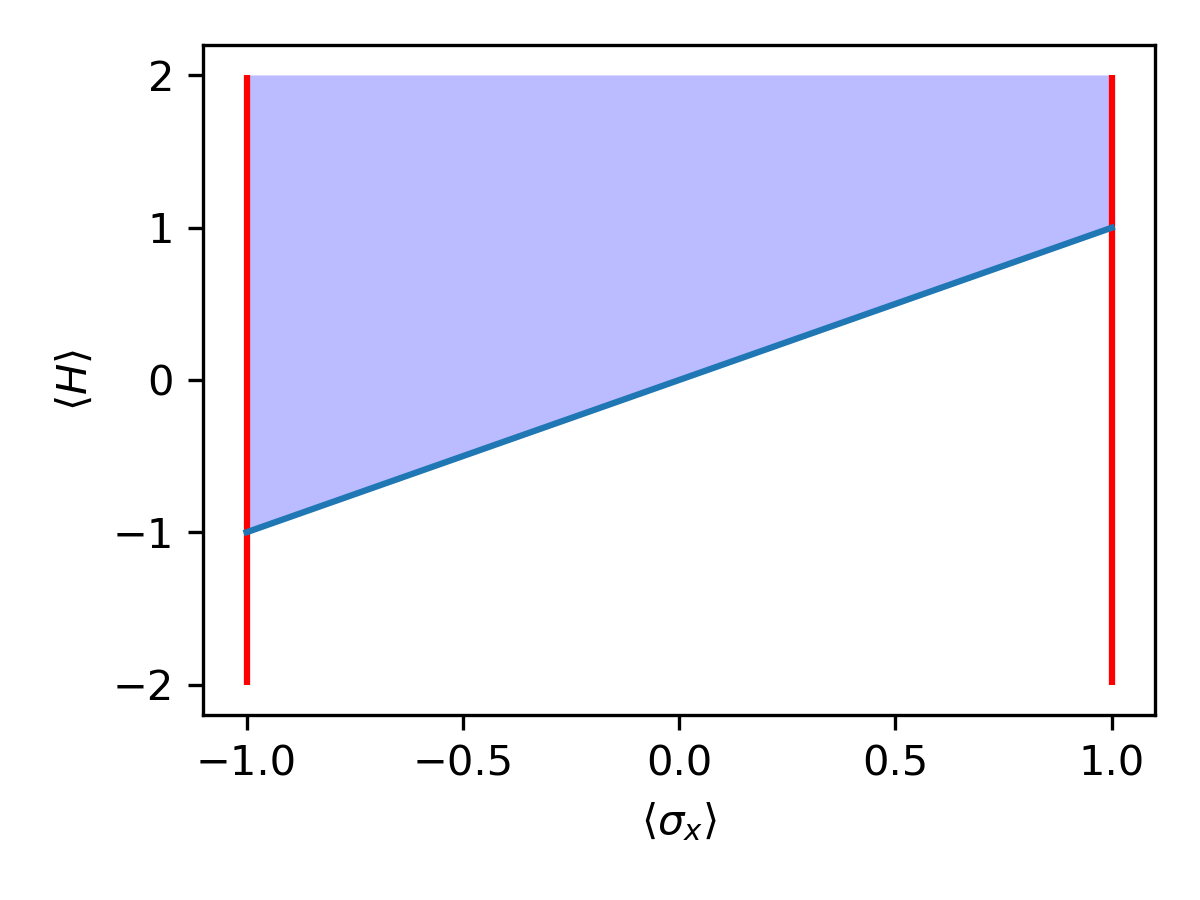# Harmonic Oscillator, Again

We can't use a complete basis. Let's start with $$\{1,x,p\}$$. $\color{green} \left(\begin{matrix} 1 & \langle x\rangle & \langle p \rangle\\ \langle x \rangle & \langle x^2 \rangle & \langle xp \rangle\\ \langle p \rangle & \langle xp \rangle + i\hbar & \langle p^2 \rangle \end{matrix}\right)\succeq 0$

This is just one small part of the "full" SDP, but it represents several important constraints!

Using the lower $$2\times 2$$ minor: $\left|\begin{matrix} \langle x^2 \rangle & \langle xp \rangle\\ \langle xp \rangle + i\hbar & \langle p^2 \rangle \end{matrix}\right| = \langle x^2 \rangle \langle p^2\rangle - \langle xp\rangle^2 - i \hbar \langle xp \rangle \ge 0 \Longrightarrow \langle x^2\rangle \langle p^2\rangle \ge \hbar$

Adding more operators can only make the bound stricter.

# Noncommutative Sum-of-Squares

$H = -\mu \sigma_x = \color{green}\frac 1 2 (1+\sigma_x)(1+\sigma_x) - 1$

From which we see that $$\color{blue}\langle H \rangle \ge -1$$

$H = \frac 1 2 x^2 + \frac 1 2 p^2 = \color{green}a^\dagger a + \frac 1 2$

Similarly, $$\color{blue}\langle H \rangle \ge \frac 1 2$$

In general, if we can write $H = C + \mathcal O_1^\dagger \mathcal O_1 + \mathcal O_2^\dagger \mathcal O_2 + \cdots$

then the bound $$\color{blue}\langle H \rangle \ge C$$ follows immediately.

This is equivalent to the semi-definite program formulation.

# Summary

• The space of permitted expectation values is convex
• The ground state sits at the boundary (the lowest possible value of $$\langle H \rangle$$)
• Any sum-of-squares representation yields a rigorous lower bound
• Equivalently, solving a truncated SDP yields a rigorous lower bound

Coming up:

Quantum information

Conformal bootstrap: a history lesson

Computational applications (matrix models, lattice field theories)

# Intermission: Quantum Information

Setup: Alice samples a random number $$i \in 1\ldots N$$, with probability $$p_i$$. Alice sends a quantum state $$\rho_i$$ to Bob. Bob must make a measurement and guess $$i$$.

How well can Bob do?

Bob will measure onto some set of projectors $$\{E_j\}$$, and reply $$j$$ with probability $P_j(|\psi\rangle) = \mathrm{Tr}\; E_j \rho_i$ We want to maximize the success probability, that is $\sum_i p_i \mathrm{Tr}\; \rho_i E_i$ subject to $E_i \succeq 0 \text{ and } \sum_i E_i = 1$

Surprise! It's an SDP!

# Intermission: The NPA Hierarchy

Alice and Bob each have access to one half of a quantum system. Alice can measure operators $$E_\alpha$$, and Bob $$E_\beta$$. A quantum correlation is: $P_{\alpha\beta} = \mathrm{Tr}\;E_\alpha E_\beta \rho$

Which probabilities $$P_{\alpha\beta}$$ are achievable quantum correlations?

Answer: $$\Gamma_{ij} = \mathrm{Tr}\; S_i^\dagger S_j \rho \succeq 0$$. So, we require that such a $$\Gamma$$ exists, compatible with the given probabilities (a linear condition). $\color{green} \mathcal S_1 = \{E_\alpha\} \cup \{E_\beta\} \;\text{ ; }\; \mathcal S_2 = \{E_\mu E_\nu\} \;\text{ ; }\; \cdots$

Tsirelson's problem: can every quantum correlation be approximated by a system of finite Hilbert space dimension?

No (!)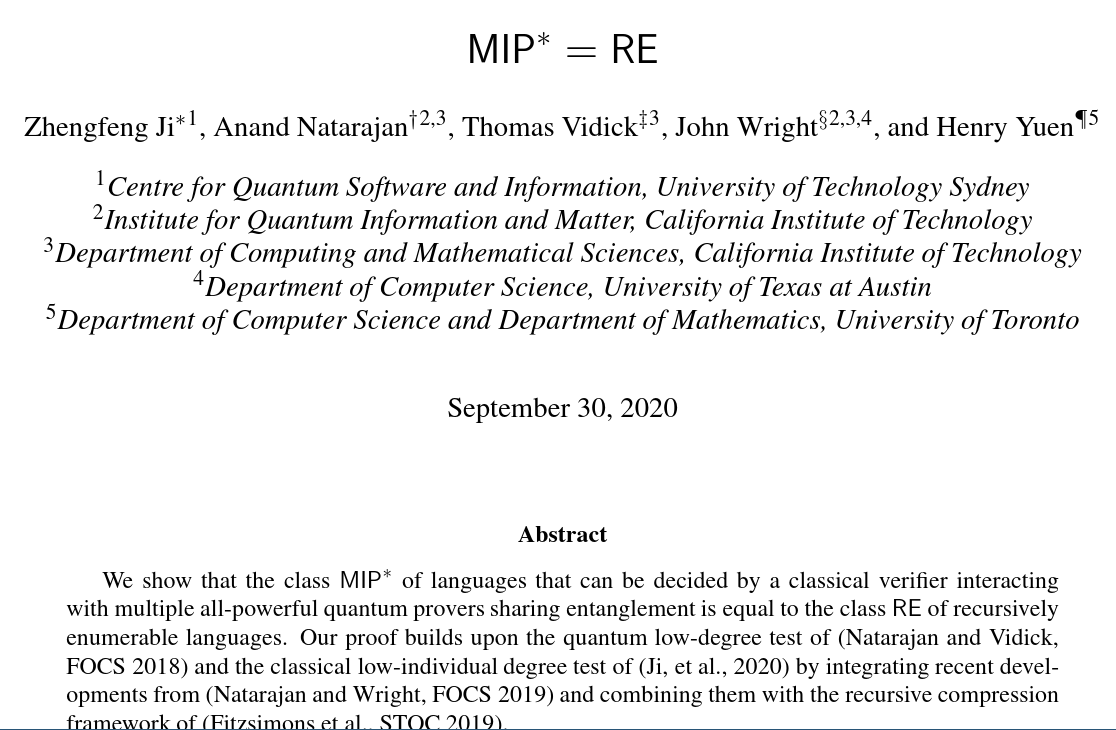# Why Does Convexity Matter?

Finding a global minimum is hard, in general.For convex functions, it's much easierCurrent tools for solving SDPs: SDP-A, MOSEK, SDPB

There is certainly room for improvement in this space.

# A Bit of History

The original dream: construct an S-matrix purely from symmetries and consistency conditions.

Circumvents the need for a sensible definition of field theory.

Fell out of favor as QCD became understood.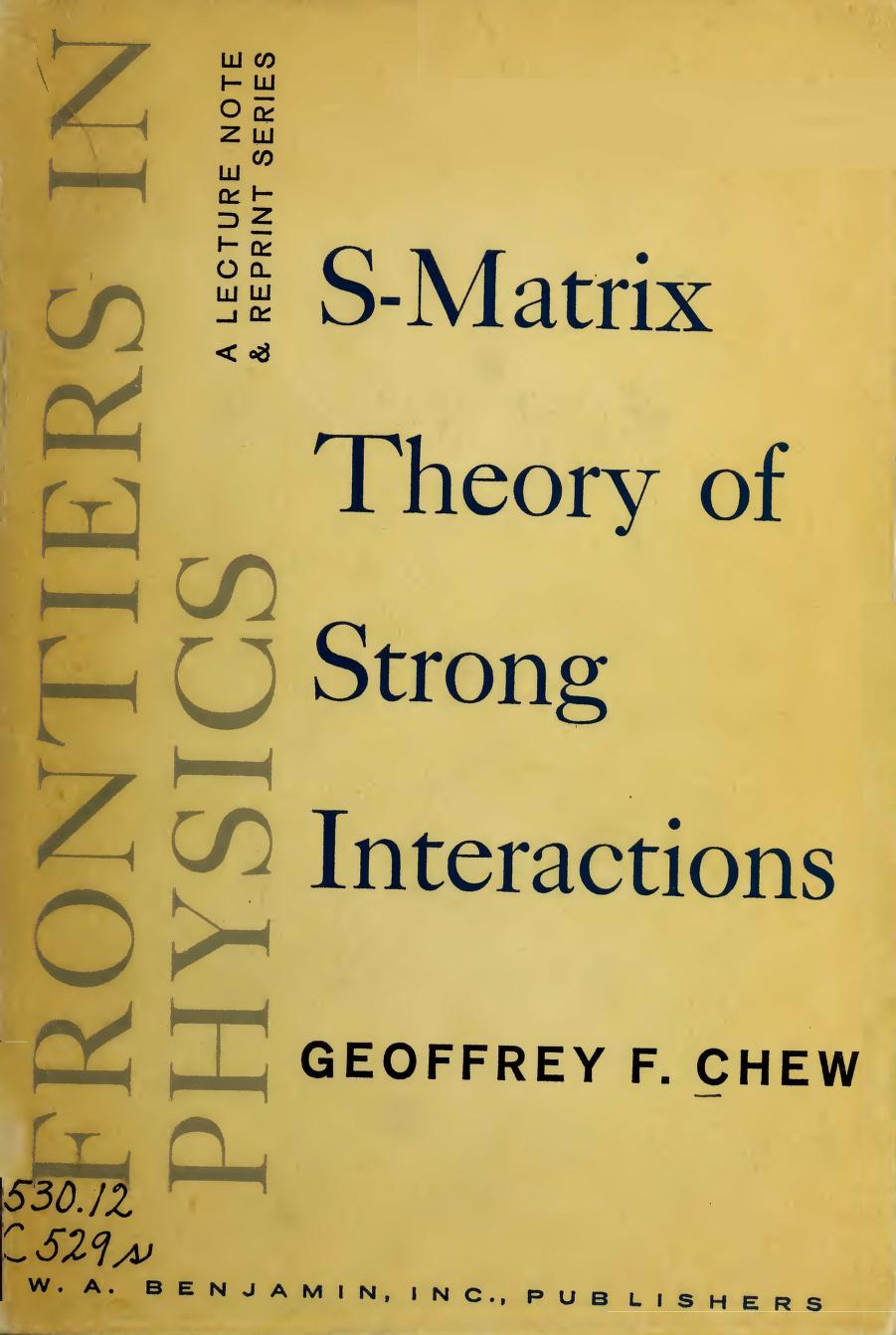$$\sim 40$$ years pass...

Conformal bootstrap: modern numerical techniques allow the bootstrap program to strongly constrain the space of conformal field theories, purely from symmetries and consistency.

Now "bootstrap" and "semi-definite programming" are somewhat synonymous.

# Conformal Field Theories

A conformal field theory is:

• Lorentz invariant
• Unitary
• Scale invariant (conjecturally implies conformal invariance)
• Characterized by critical exponents

Why do we care?

At asymptotically high or low energies, any field theory approaches a conformal fixed point.

Also, lamppost effect: we study these because they're (relatively) easy.

# Conformal Bootstrap

• Conformal invariance
• Crossing symmetry
• Unitarity $$\Rightarrow$$ Radially quantized states have positive norm
• Internal symmetries ($$\mathbb Z_2$$)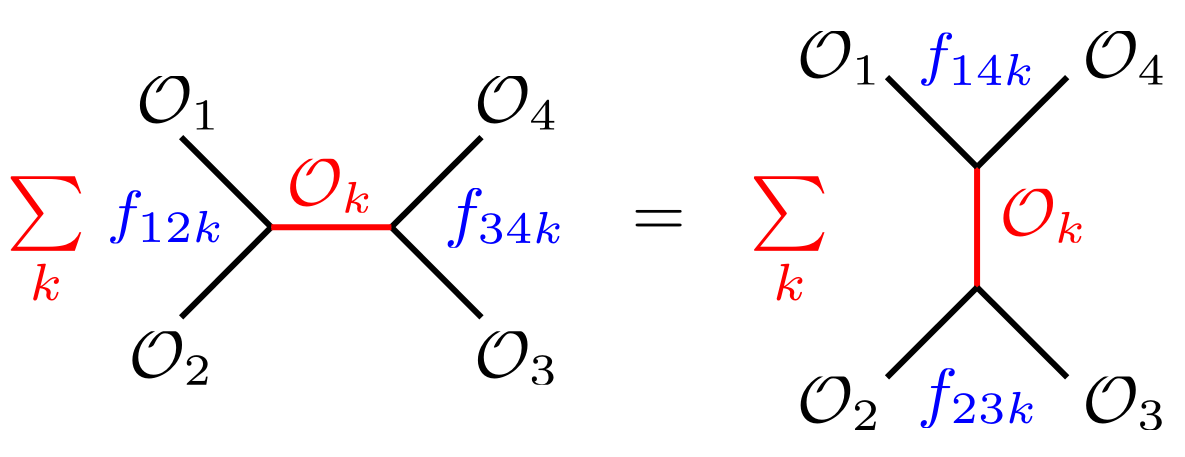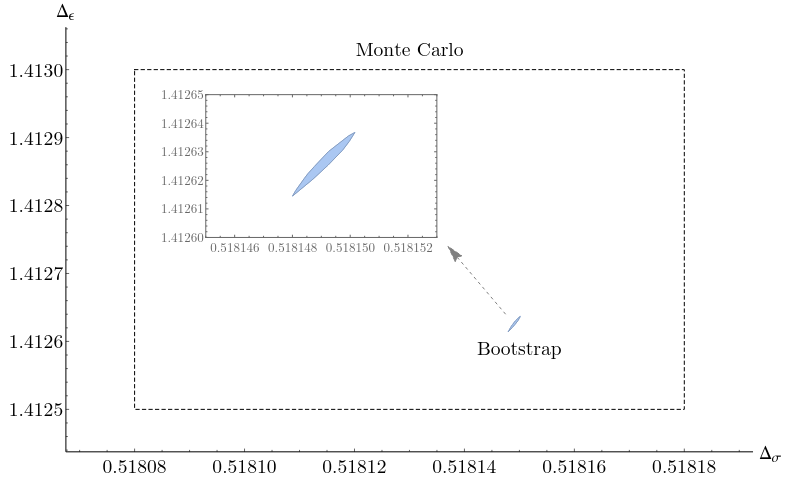# Finding Eigenvalues

To study individual eigenstates, we can impose: $\langle H \mathcal O \rangle = E \langle \mathcal O \rangle$

For the anharmonic oscillator, this yields constraints like: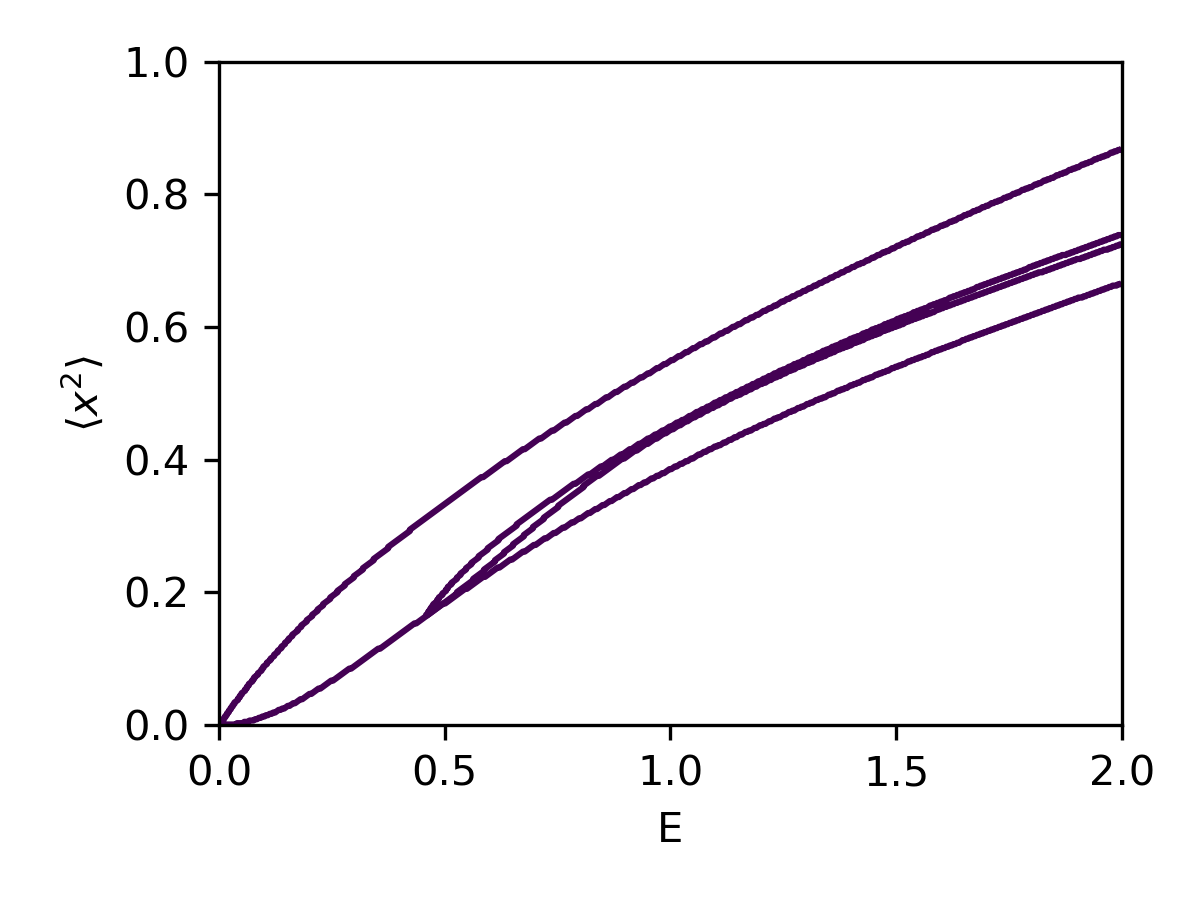The allowed region is not convex!

# Matrix Models

Let $$X,P$$ be $$N\times N$$ Hermitian matrices of operators, obeying $[P_{ij},X_{kl}] = -i \delta_{ik} \delta{jl}$

Some Hamiltonians $\color{green} H_{\mathrm{easy}} = \mathrm{Tr}\; P^2 + \mathrm{Tr}\;X^2 + \frac{g}{N}\mathrm{Tr}\;X^4$ $\color{red} H_{\mathrm{harder}} = \mathrm{Tr}\; P_X^2 + \mathrm{Tr}\; P_Y^2 + m^2 \mathrm{Tr}\;(X^2 + Y^2) - \mathrm{Tr}\;g^2[X,Y]^2$

Matrix models are quantum mechanical and consistent.

Conjecture: Larger matrix models (e.g. BFSS) exhibit emergent spacetime.

# Bootstrapping Matrix Models

$\color{green} H_{\mathrm{easy}} = \mathrm{Tr}\; P^2 + \mathrm{Tr}\;X^2 + \frac{g}{N}\mathrm{Tr}\;X^4$
$\langle \mathrm{Tr} \left(\begin{matrix} 1 & X^2 & 0 & 0\\ X^2 & X^4 & 0 & 0\\ 0 & 0 & X^2 & XP\\ 0 & 0 & PX & P^2\\ \end{matrix}\right)\rangle \succeq 0$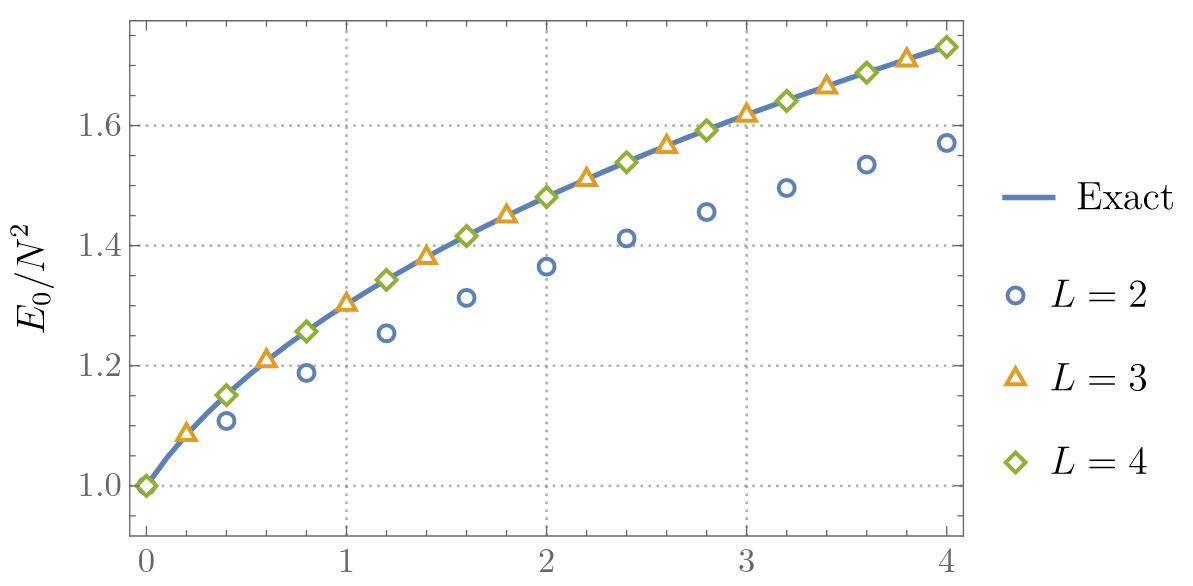$\color{red} H_{\mathrm{harder}} = \mathrm{Tr}\; P_X^2 + \mathrm{Tr}\; P_Y^2 + m^2 \mathrm{Tr}\;(X^2 + Y^2) - \mathrm{Tr}\;g^2[X,Y]^2$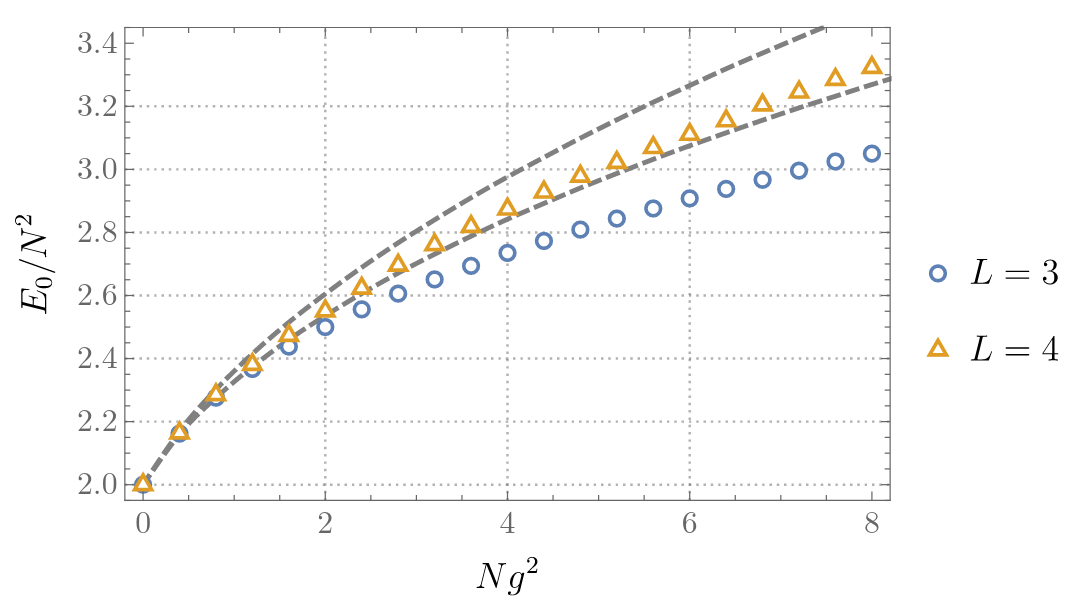# Lattice Field TheoryEach lattice site has a degree of freedom with Hilbert space $$\mathcal H_1$$. The whole system has Hilbert space $$\mathcal H = \mathcal H_1 \otimes \mathcal H_1 \otimes \cdots$$

Some Hamiltonian $$H$$ couples the different lattice sites. For a spin system, we might have $$H = \sum_{\langle i j\rangle} \sigma_z(i) \sigma_z(j) + \sum_i \sigma_x(i)$$

When correlations are large, the lattice structure is irrelevant, hence "field theory".

# The Bootstrap on the Lattice

$H = \sum_x \left[\frac 1 2 \pi(x)^2 + \frac 1 2 (\phi(x) - \phi(x-1))^2 + \frac{m^2}{2} \phi(x)^2 + \lambda \phi(x)^4\right]$

With $$m = 0.2$$

Operators:

• $$1,\pi(0)$$
• $$\phi(0),\phi(1),\ldots,\phi(L)$$
• $$\phi(0)^2,\phi(0)^3,\ldots,\phi(0)^N$$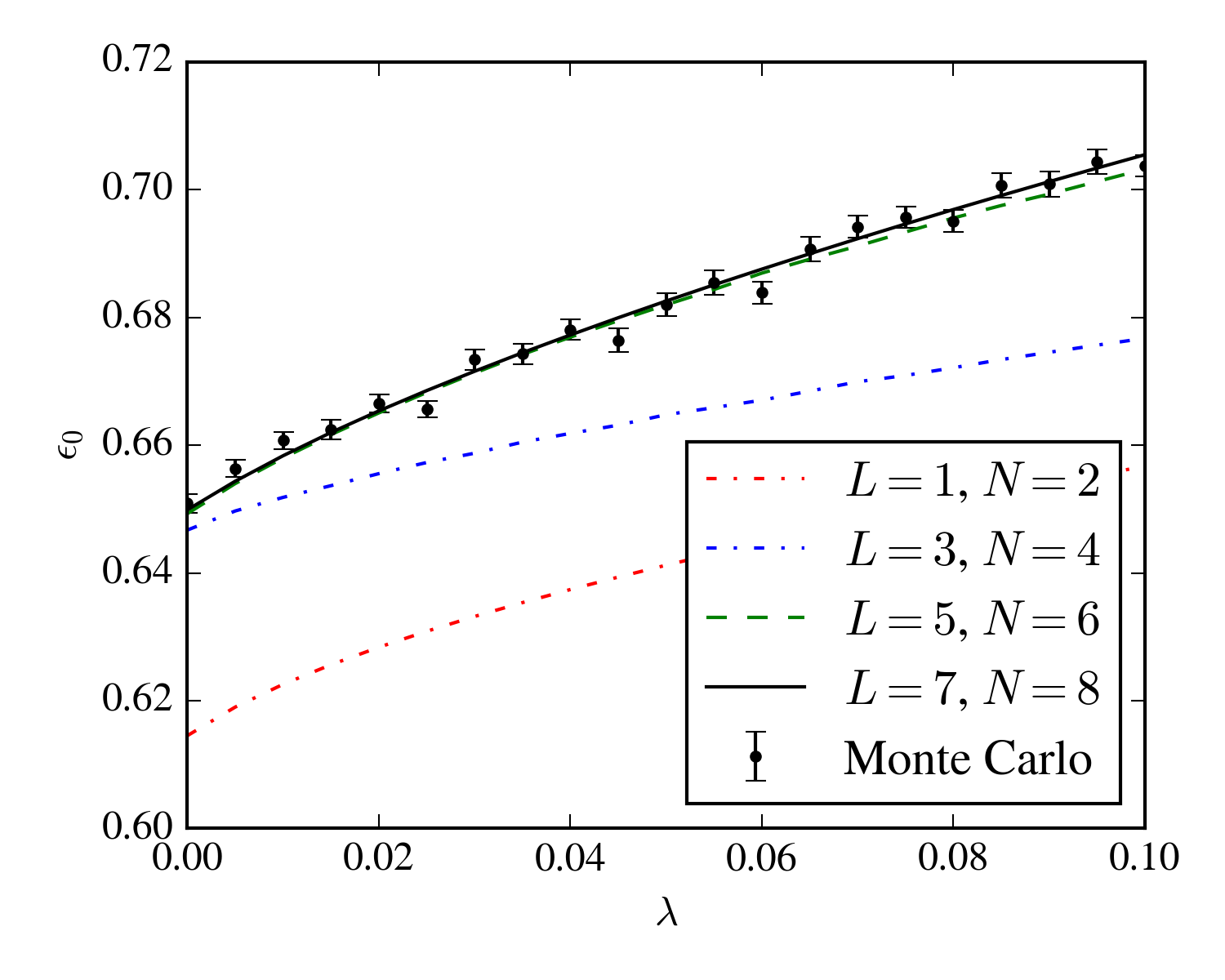# A Hard Problem: Finite Density Fermions

Monte Carlo (i.e. lattice QCD) methods are powerful, but fail for relativistic theories at finite fermion (proton - antiproton) density.

First noted in 1990. Not well-understood in general.

Makes it difficult to study:

• Superconductors (and other strongly interacting electrons)
• Large nuclei
• Dense nuclear matter (neutron stars)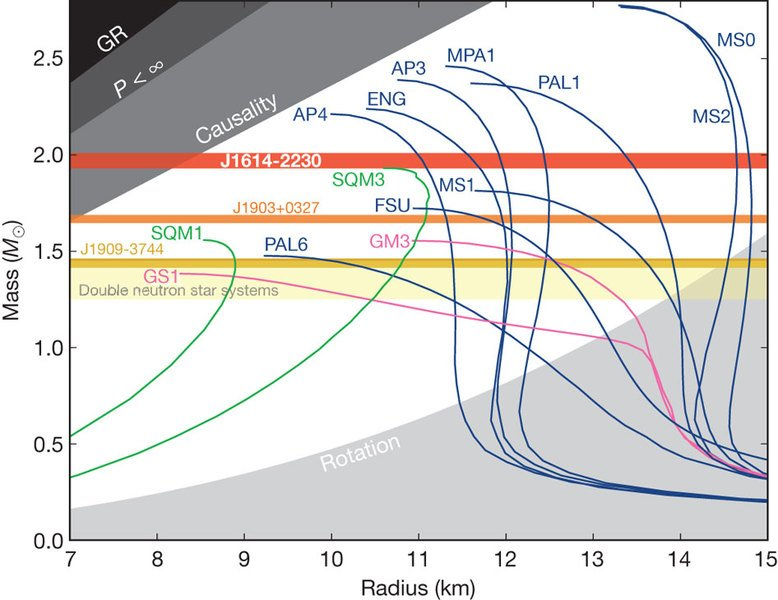# Bootstrapping Fermions

The lattice Thirring model (staggered fermions):

$H = \sum_x (-1)^x m \chi^\dagger(x) \chi(x) + {\color{red} \mu \chi^\dagger(x) \chi(x)} + (-1)^x\frac{\chi^\dagger(x) \chi(x+1) + \mathrm{h.c.}}{2} +g^2 \chi(x) \chi^\dagger(x)\chi^\dagger(x+1)\chi(x+1)$ $\text{ where }\; \{\chi(x),\chi(y)\} = 0 \text{ and } \{\chi(x)^\dagger,\chi(y)\} = \delta_{xy}$

At finite chemical potential, lattice Monte Carlo exhibits the sign problem.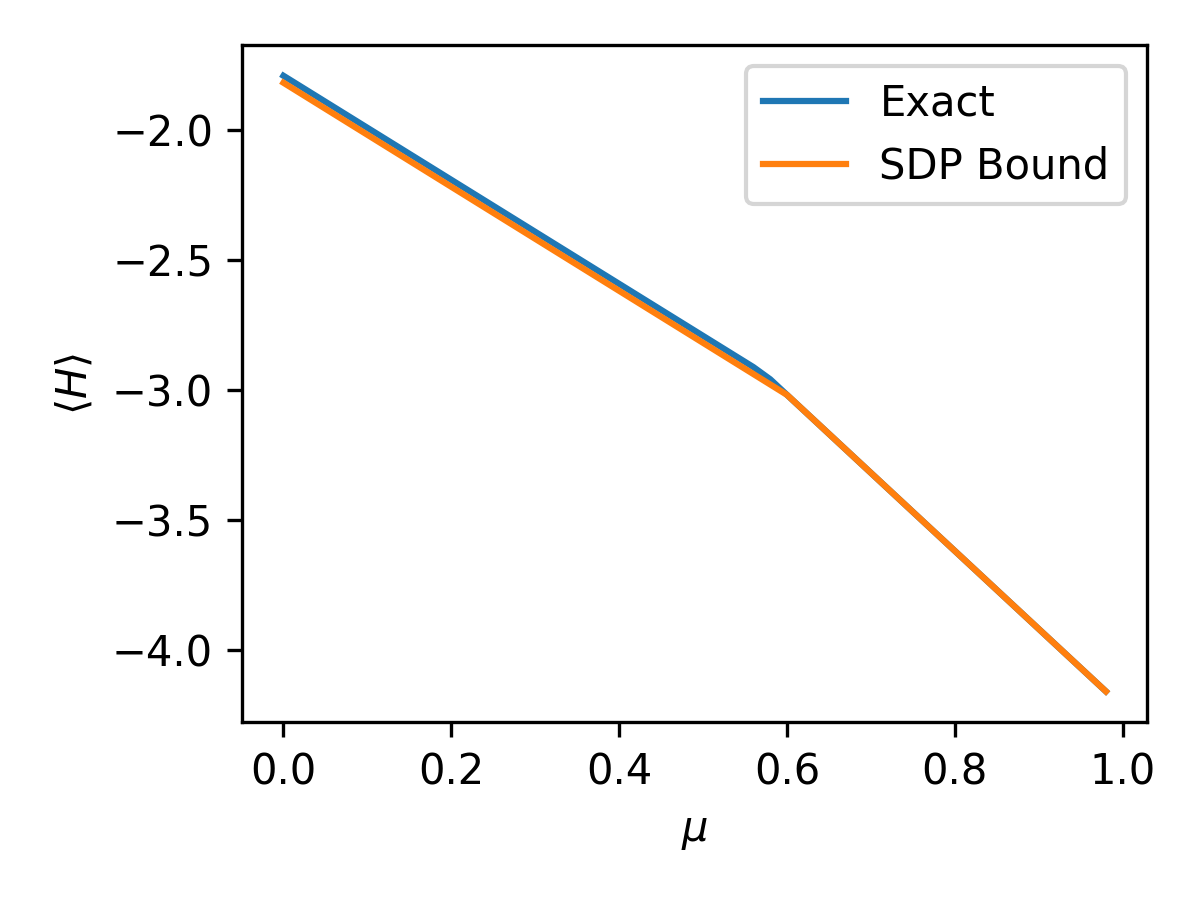• Operators: all $$\chi(x)$$ and $$\chi^\dagger(x)$$
• $$L = 4$$
• $$m = 0.5$$
• $$g^2 = 0.1$$

No evidence of a sign problem

# Open Problems

Direct extensions
• QM fermions
• Continuum limit
• Finite temperature
• Time-separated correlation functions
• Better tools. Faster, easier to use.
• Understand scaling:
• For QM bootstrap, getting $$d$$ digits takes polynomial time, empirically. Why?
• For field theories, it's unknown
• Why is the conformal bootstrap so successful?
• How do sign problems manifest?
• Find other worthy targets
FIN
/
< >